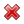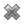# Fight Finance

#### CoursesTagsRandomAllRecentScoresScoreskeithphw $6,011.61 Jade$1,815.80 ZOE HY $860.33 Chu$789.98 royal ne... $750.00 Leehy$713.33 Visitor $650.00 JennyLI$625.61 Visitor $590.00 Visitor$555.33 Visitor $550.00 Visitor$550.00 Visitor $540.00 Visitor$500.00 Yizhou $489.18 Visitor$480.00 Visitor $480.00 Visitor$470.00 Visitor $464.70 Visitor$460.00

A stock is expected to pay the following dividends:

 Cash Flows of a Stock Time (yrs) 0 1 2 3 4 ... Dividend (\$) 0.00 1.15 1.10 1.05 1.00 ...

After year 4, the annual dividend will grow in perpetuity at -5% pa. Note that this is a negative growth rate, so the dividend will actually shrink. So,

• the dividend at t=5 will be $1(1-0.05) = 0.95$,
• the dividend at t=6 will be $1(1-0.05)^2 = 0.9025$, and so on.

The required return on the stock is 10% pa. Both the growth rate and required return are given as effective annual rates.

What is the current price of the stock?